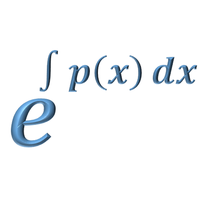# Differential Equations - Integrating FactorOn this page we will look at another type of differential equations: linear differential equations in the form y' + P(x)y=Q(x) which can be solved by using an integrating factor. There are many applications of this type of differential equation....

To access the contents of this site, you need to log in or subscribe